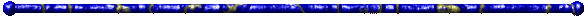# Stupid-Site

## DrStupid's Linear Regression : y(x) ≈ Σ pi·fi(x)data: xk; yk; wk functions: fi(x) parameters: pi

flip x and y    ignore weight factors

How to use?

Enter source data in two rows (x and y) or three
rows (x, y and weight factor) separated with Tab or ";".
You may copy and past data directly from EXCEL.
Missing weight factors will be set to 1.
"." and "," will be accepted as decimal separator.

Example:
```1        1.0        1
2; 2,0
```

For approximation, data can also be generated by input
of a function and a range for the x values.

Example:
```sin(x)
0 ; 6.28 ; 100
```
generates 100 data points with x ranging from 0 to 6.28
and y=sin(x)

How to use?

Enter one function fi(x) of
the linear combination per Line.
You may use all mathematical
operators and Math functions
supported by Javascript.

Example (linear regression):
```1
x

```